## All About These 15 Worksheets

These Decimal Addition worksheets are designed to help students master the concept of adding decimal numbers. The worksheets include a variety of creative and engaging exercises to help students build their skills and confidence in decimal addition.

Each worksheet in the series includes a mix of problems with varying degrees of difficulty, designed to challenge and engage students at different skill levels. The problems require students to add decimal numbers with different numbers of decimal places and to carry over decimals when necessary.

This series is most suitable for students in grades 5 to 7. It can be used in the classroom as a supplement to the math curriculum or at home as a way for parents to help their children practice and reinforce their math skills.

Overall, these Decimal Addition worksheets provide a comprehensive and engaging way for students to develop their skills and confidence in adding decimal numbers. With these worksheets, students can build a solid foundation in math and develop the skills they need to succeed in higher-level math courses.

## How to Add Decimal Values

Adding decimal values is similar to adding whole numbers, with the added step of aligning decimal points. Here’s a step-by-step guide to adding decimal values that teachers can share with their students:

1. Align Decimal Points – Write the numbers vertically so that the decimal points line up with each other. This makes it easier to add digits in the same place value.
2. Add Zeros, if Necessary – If one decimal has more digits after the decimal point than the other, add zeros to the shorter decimal so that they have the same number of digits. This will make adding the decimals simpler. Remember that adding zeros to the right of a decimal number does not change its value.
3. Start Adding From the Rightmost Digit – Begin with the rightmost digits (the smallest place value) and add them together. If the sum is 10 or greater, carry the 1 to the next place value on the left.
4. Continue Adding Digits – Continue adding the digits in each place value from right to left, carrying any value of 10 or greater to the next place value on the left.
5. Place the Decimal Point – Once you have added all the digits, place the decimal point in the sum directly below the aligned decimal points of the numbers being added.
6. Simplify, if Necessary – If the sum has trailing zeros after the decimal point (e.g., 4.50), you can remove them to simplify the result (e.g., 4.5).

Here’s an example of adding 3.25 and 0.879:

Step 1: Align decimal points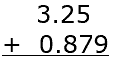Step 2: Add zeros, if necessary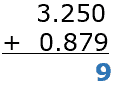Start adding from the rightmost digit: 9 (0+9)

Step 3: Continue adding digits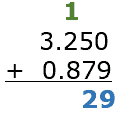12 (5+7, carry the 1)

Step 4: 11 (1 + 2 + 8, carry the 1)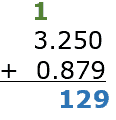Step 5: Place the decimal point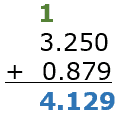Simplify, if necessary: No trailing zeros in this case, so the sum remains 4.129.

The sum of 3.25 and 0.879 is 4.129.

## How to Master Decimal Addition

Learning to add decimals is an essential skill for students, and it can be taught effectively using a step-by-step approach. Here’s a suggested process for teaching students how to add decimals:

• Ensure understanding of decimals – Before students begin learning to add decimals, make sure they have a solid understanding of decimals and their place values (tenths, hundredths, etc.). They should also be comfortable with the concept of fractions and how they relate to decimals.
• Review basic addition – Students should have a strong foundation in basic addition with whole numbers before attempting to add decimals.
• Align the decimals – Teach students that when adding decimals, it’s crucial to align the decimal points in a vertical arrangement. This ensures that each place value lines up correctly. Instruct students to write one number below the other, aligning the decimal points, and then draw a horizontal line below the numbers.
• Add zeros if necessary – To make it easier to add decimals with different numbers of decimal places, students can add zeros to the end of the shorter decimal number. This ensures that each decimal place has a corresponding number to add.
• Add from right to left – Students should start adding the numbers from the right-most column (smallest place value) and move to the left, just as they would with whole numbers. They should carry over any values when necessary.
• Place the decimal point – In the final answer, the decimal point should be placed directly below the decimal points in the original numbers, maintaining its position relative to the place values.
• Practice with examples – Provide students with numerous examples of decimal addition problems. Start with simple problems and gradually increase the difficulty level as they become more comfortable with the process.
• Use manipulatives or visual aids – To help students grasp the concept of adding decimals, use manipulatives like base-ten blocks or grid paper to visually represent the addition process.
• Incorporate real-life scenarios – Encourage students to apply their decimal addition skills to real-life situations, such as calculating prices, measuring distances, or working with time. This helps them understand the practical applications of decimal addition and reinforces their learning.
• Review and practice regularly – Ensure that students regularly review and practice adding decimals to build their confidence and fluency.

By using this step-by-step approach and incorporating a variety of teaching methods, students can effectively learn to add decimals and develop a strong foundation in this essential mathematical skill.